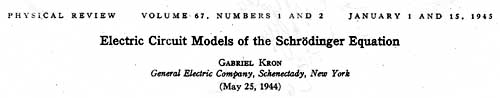{This website: Please note: The following article is complete; it has been put into ASCII due to a) space requirement reduction and b) for having thus the possibility to enlarge the fairly small figures. And: special math. symbols are in Unicode. Further: disallow M\$-IE 'automatic picture size adaptation'.}

Gabriel Kron's biography here.

Abstract: Equivalent circuits are developed to represent the Schrödinger amplitude equation for one, two, and three independent variables in orthogonal curvilinear coordinate systems. The networks allow the assumption of any arbitrary potential energy and may be solved, within any desired degree of accuracy, either by an a.c. network analyzer, or by numerical and analytical circuit methods. It is shown that by varying the impressed frequency on a network of inductors and capacitors (or by keeping the frequency constant and varying the capacitors), it is possible to find by measurements the eigenvalues, eigenfunctions, and the statistical mean of various operators belonging to the system represented. The electrical model may, of course, be replaced by an analogous mechanical model containing moving masses and springs.

At first the network for the one-dimensional wave equation for a single particle in Cartesian coordinates is developed in detail, then the general case. A companion paper contains results of a study made on an a.c. network analyzer of one-dimensional problems: a potential well, a double barrier, the harmonic oscillator, and the rigid rotator. The curves show good agreement, within the accuracy of the instruments, with the known eigenvalues, eigenfunctions, and "tunnel" effects.

THE ONE-DIMENSIONAL SCHRÖDINGER EQUATION

In Cartesian coordinates, the equation is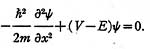Three types of equivalent circuits may be established.

1. The circuit contains positive and negative resistors and in each state the currents and voltages are constant in time. The state is changed by varying the resistances, corresponding to a change in eigenvalue (energy level).

2. Although negative resistances are available for use with a network analyzer, in practice it is more convenient to use a second type of circuit, in which the positive and negative resistors are replaced by inductors and capacitors and the d.c. currents and voltages are replaced by a.c. currents and voltages of fixed frequency. The use of the second type of interpretation is equivalent to multiplying the wave equation by i = √- 1.

In the diagrams to follow, unless otherwise stated, the inductors (whose reactance at the fixed frequency is denoted by XL ) may also be viewed as positive resistances of value XL and the capacitors (whose reactance is denoted by - XC ) as negative resistances of value - XC.

3. The third type of circuit contains inductors and capacitors and in each state the currents and voltages are sinusoidal in time. The state is changed by varying the frequency of the impressed voltage.

The basic network concepts will be introduced in detail in connection with the first type of circuit (with additional comments on the second type of interpretation). The third type of model, although it offers attractive analogies, will be only cursorily treated.

REPRESENTATION OF ENERGY OPERATORS

If the wave equation is multiplied by ∆x and the operator p = - i ħ∂ /∂x is introduced, the equation becomes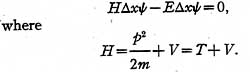The three energy operators will be represented in the following manner: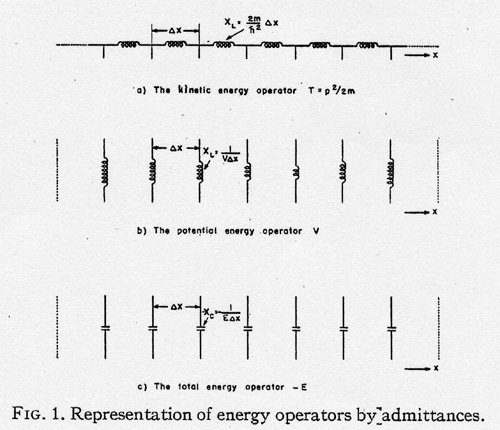1. The kinetic energy operator T = p2/ 2m is represented (Fig. 1a) by a set of equal positive resistors (or inductive coils) in series extending from -∞ to + ∞. The impedance of each coil is (2m/ ħ2)∆x.

2. The potential energy operator V is represented (Fig. 1b) by a set of isolated positive resistors (inductive coils). The admittance of each coil is V∆x. These values vary from point to point.

3. The total energy operator -E is represented (Fig. 1c) by a set of isolated equal negative resistors (capacitors). The admittance of each coil is the unknown quantity E∆x.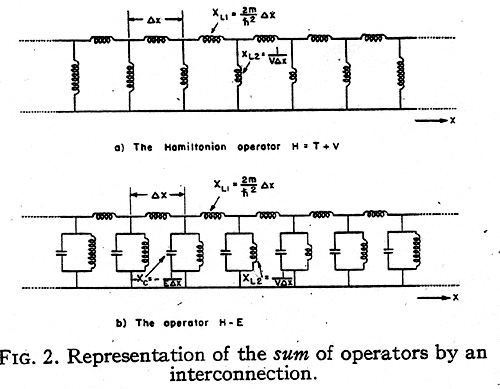The sum of the two operators T and V forming the Hamiltonian operator H is represented by the interconnected network of Fig. 2a. That is, a summation of energy operators is represented electrically by an interconnection of the component networks.

Finally, the sum of the Hamiltonian H and the total energy -E operators is the resultant network of Fig. 2b extending from - ∞ to + ∞.

OPERATION ON THE WAVE FUNCTION ψ

The wave function ψ will be assumed to be represented (Fig. 3) by the differences of potential (1) appearing between the junctions of the three types of coils and the ground connection (impedanceless wire). The function ψ varies along ψ' but is constant in time. An eigenfunction ψ' of H depends on the time as follows: ψ' = ψ exp [-i(E/ ħ)t].

The differences of potentials appearing between two junctions are ∆ψ = (∂ψ/ ∂x )∆x + ... where higher order terms in the Taylor series development are neglected.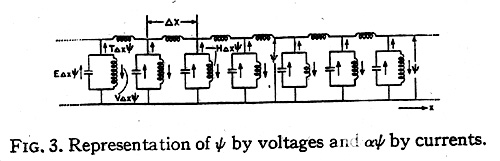The result of the operation αψ or βψ (where α and α are operators) is represented by currents flowing in the respective component networks, as shown in Fig. 3. In particular:

1. The currents flowing in the capacitors are E∆xψ.

2. The currents flowing in the vertical inductors are V∆xψ.

3. The currents flowing in the horizontal inductors are β∆ψ = (ħ2/ 2m)(∂ψ/∂x).

4. The currents flowing out of the horizontal inductors at their junctions are
∆(β∆ψ) = (ħ2/2m)(∂2ψ/∂x2) representing (-T∆xψ).

Since E∆xψ represents the currents flowing in the capacitors and H∆xψ = (T+ V)∆xψ those in the inductors, the equation H∆xψ = E∆xψ simply states Kirchhoffs second law, that at each of the junctions of four coils the currents flowing into the positive resistors (inductors) are equal to the currents coming from the negative resistors (capacitors).

Both the voltages and currents appear as standing waves. When the space distribution of ψ is such that it attenuates, the network may be terminated at any point by an equivalent impedance. When ψ does not attenuate the circuit may be terminated by a short circuit at any point of zero voltage, or by an open circuit at any point of zero current. These points are easily determined on the a.c. network analyzer by trial.

It should be noted that the equivalent circuit introduces an indeterminacy between quantities measured in the vertical coils and those in the horizontal coils. If, for instance, the vertical voltage ψ is known at a certain value of x, say x0, then the horizontal current (ħ2/2m)(∂ψ/∂x) is not known at that particular x0, only at some indeterminate value between x0 and x0+∆x/2 or x0-∆x/2.

EIGENVALUES AND EIGENFUNCTIONS

Let it be assumed as an example that the V function is a potential well (Fig. 4a). Then in the network the corresponding positive resistances assume either a constant or a zero value as shown in Fig. 4b. In practice it is sufficient to extend the network to, say, twice the width of the potential well on either side.

Let now a d.c. (or a.c.) generator be inserted anywhere in the network parallel with one of the negative resistances (inductors), as shown. If the values of all the negative resistances -E∆x (capacitors) are simultaneously varied by the same amount, it will be found that the current (reactive current) in the generator varies and at some value of E∆x becomes zero.

It should be noted that while a current (reactive current) flows in the generator the circuit does not satisfy the differential equation, since at one point in the network (where the generator is connected) the currents do not add up to zero (as required by the equation) but to the generator current. That is, while a current flows in the generator, the voltages ψ do not represent solutions of the differential equation. Hence, only those network conditions are of interest in which the generator current is zero.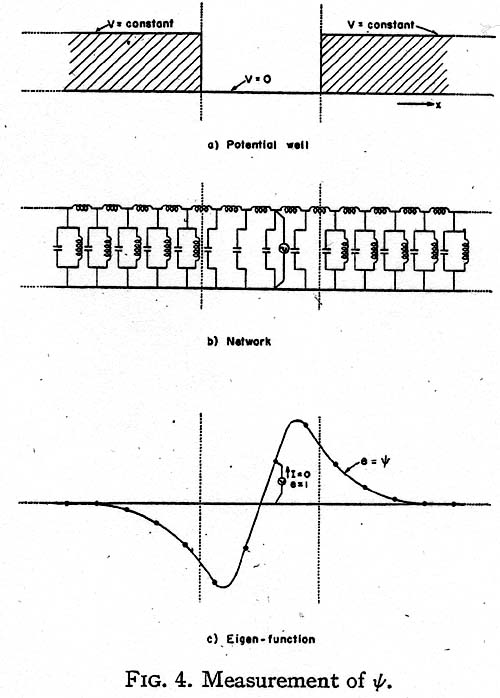Now a value E of the negative resistances, at which the generator current becomes zero, represents a state at which the circuit is self-supporting and has a continuous existence of its own without the presence of the generator, as the negative resistances just supply the energy consumed by the positive resistances. (If the circuit contains inductors and capacitors, the circuit is a resonant circuit and it oscillates at its basic frequency.) E is then an eigenvalue En, while the voltage distribution across the capacitors (Fig. 4c) gives the corresponding eigenfunction ψn.

When the generator current is positive the circuit draws energy from the source, and when the current is negative the circuit pumps back energy into the source. At zero generator current the circuit neither gives nor takes energy, and theoretically the generator may be removed. All values of E at which the current crosses the axes and becomes zero are eigenvalues of the equation and the corresponding voltage distribution curves are eigenfunctions. When the energy level E overflows the well, the discrete spectrum of eigenvalues changes into a continuous spectrum and the generator current is zero at all greater values of E.

When the energy E changes sign, the negative resistances become positive resistances and at no value of -E may the circuit be self-supporting (as it contains only positive resistances). That is, the equation has no negative eigenvalues.

THE STATISTICAL MEAN OF OPERATORS

To bring the measurements at the different energy levels to the same base, it is necessary to normalize the measured ψ values so that the new values of ψ satisfy the equation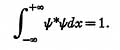The measured ψ functions are normalized by plotting the square of ψ. If the area under the curve is 1/N2, all values of ψ are multiplied by N. Then Nψ is the normalized ψ. (Actually N may contain an arbitrary phase e .)

The statistical mean of an operator α for a state ψ is defined as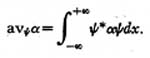Since (a∆x)ψ is a current (reactive current) flowing through an admittance, ψ*(a∆x)ψ is the power (reactive power) in a single admittance. Hence the total power in a complete set of similar admittances, namely,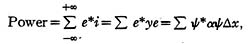represents the statistical mean of the corresponding energy operator. That is:

1. The total power in all the vertical negative resistors is the average value of E. (That is, E itself, since ψ is an eigenfunction of H).

2. The total power in all the vertical resistors is the average value of the potential energy V.

3. The total power in all the horizontal resistors is the same as the total power in the vertical units, representing the average of the kinetic energy T = p2/ 2m.

That is, the total power in all positive resistors is the same as the total power in all the negative resistors, or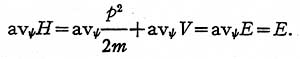THE THIRD MODEL

Let the wave equation be divided by iωc, where ωc = √ω = (E/ ħ)½, and multiplied by ∆x: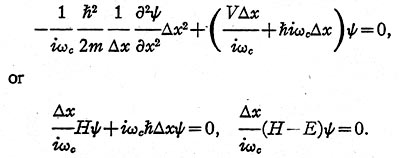In the present case:

1. The kinetic energy operator T is represented (Fig. 5) by a set of equal inductors in series, whose inductance L1 is (2m/ ħ2)∆x.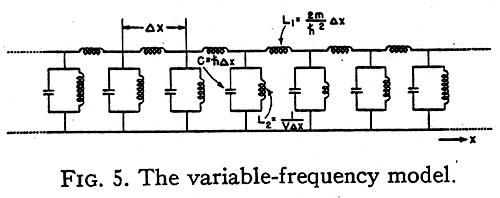2. The potential energy operator V is represented by a set of unequal coils in parallel, whose inductance L2 is 1/ V∆x.

3. The total energy operator - E is represented by a set of equal capacitors in parallel whose capacitance is now ħ∆x. (In the second model the capacitance was the unknown E∆x.)

The operand ψ is again represented by voltages and the result of the operation αψ by the same currents as in the first model. Their variation in time now is sinusoidal, with 2 π fc = ωc.

Instead of varying the magnitude of the capacitors, now the frequency of the generator is varied, thereby varying the admittance of the capacitors, ħωc = E (and those of the inductors). Again when the generator current becomes zero the circuit is oscillatory and self-supporting and the network represents a stationary solution of the equation. The corresponding eigenvalue is E = ħω = ħ(ωc )2, rather than ħωc, because of the simultaneous variation of the reactance of the inductive coils. The eigenfunctions ψ of the model and of the equation are, however, identical.

As the currents in the horizontal inductors are (ħ2/2miωc )∂ψ/ ∂x, the results of an operation on ψ by the momentum operator p = (ħ / i)∂/ ∂x are these currents divided by ħ2/ 2mωc. Hence, in the third model the momentum operator p may be represented by a set of equal horizontal coils with inductance L = ∆x/ħωc.

One slight disadvantage of this third model is that as the energy E changes sign, the reactance of the capacitor jωcħ cannot change signs. Since in most cases no eigenvalues exist in the negative energy range, this disadvantage is of little consequence. Of course, the second model with fixed frequency and variable capacitors works in all cases, since the capacitors simply become inductors when E changes sign.

THE FREE PARTICLE IN ONE DIMENSION

An interesting special case occurs when the potential V is zero everywhere. The one-dimensional equivalent circuit of such a free particle is a conventional transmission line extending to infinity in both directions (Fig. 6) in which the series inductance is 2m ∆x/ ħ2 and the shunt capacitor is ħ∆x.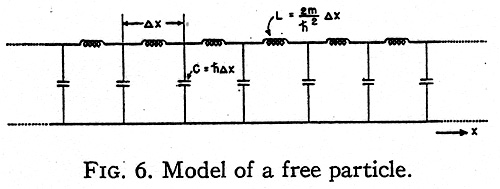It is well known that such a transmission line may maintain a standing wave at any frequency ψ=ωc between zero and infinity drawing no current from the generator. That is, the positive energy values form a continuous spectrum. If the transmission line is considered as the second type of model with variable capacitors, then at negative energy values E the capacitors also become inductors and the line cannot maintain a standing wave. The corresponding free particle also has no eigenvalue at the negative energy levels.

MODELS ALONG CURVILINEAR AXES

The three-dimensional Schrödinger equation for a single particle is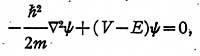where 2 is the Laplacian operator in curvilinear coordinates.

In order to establish a physical model for it, it is necessary to change it to a tensor density equation. (2)

The above equation in orthogonal curvilinear coordinates may be changed to a tensor density form by multiplying it by h1h2h3 = √g givingIf the equation is multiplied through by ∆u1∆u2∆u3, it represents the surface integral of grad ψ around the six faces of a cube of space with volume h1h2h3∆u1∆u2∆u3. The width ∆uα may be arbitrary and different in the three directions.

The corresponding equivalent circuit is shown in Fig. 7. For a free particle (V=0) it represents a generalization of the conventional one-dimen- sional transmission line to three dimensions.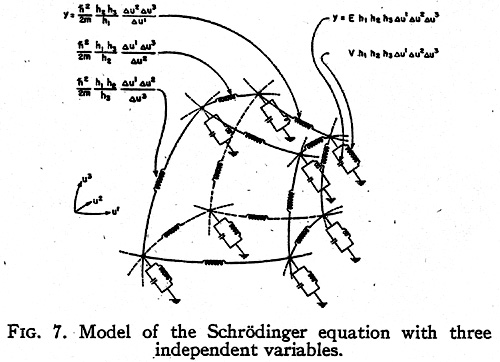NOTES AND REFERENCES

(1) It is possible to establish in one dimension a dual network in which ψ is represented by a current instead of a voltage. However, in two and three dimensions the dual networks require ideal transformers.

(2) G. Kron, Proc. I. R. E, 32, 289-299 (May, 1944).http://www.quantum-chemistry-history.com